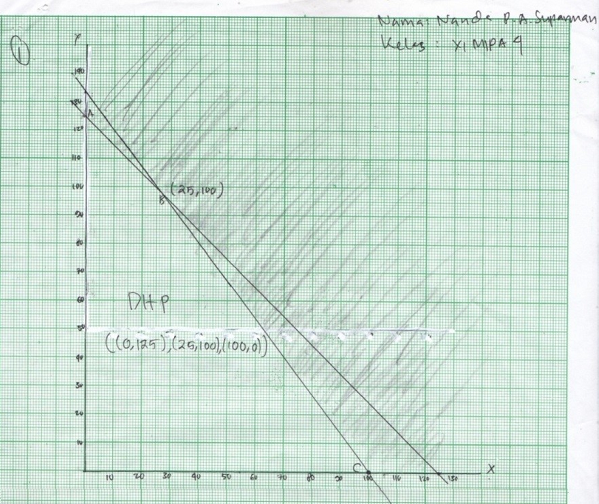# ANALISIS KEMAMPUAN REPRESENTASI MATEMATIS PESERTA DIDIK PADA MATERI PROGRAM LINEAR

• Eunike Ester Mataheru Universitas Pattimura
• Tanwey Gerson Ratumanan Universitas Pattimura
• Carolina Selfisina Ayal
Keywords: mathematical representation ability, linear programming

### Abstract

Mathematical representation ability is the ability to present notations, symbols, tables, pictures, graphs, diagrams, equations, or other mathematical expressions into other forms possessed by students to find solutions to a problem at hand. Therefore, teachers should provide sufficient opportunities for students to be able to train and develop mathematical representation skills as an important part of solving the problems given. The mathematical representation ability of students is still low. Students still find it difficult to solve mathematical problems related to aspects of verbal indicators, picture indicators, and symbol indicators. This can be found in linear programming material. Also, linear programming material is often considered difficult material by students in learning mathematics. This study aims to describe students' mathematical representation abilities including symbol representation, image representation, verbal representation on linear programming material in class XI SMA Negeri 3 Ambon. The type of research used is combination research (mixed methods). The research was conducted at SMA Negeri 3 Ambon with 36 research subjects from class XI MIPA 4 and the subjects chosen to be interviewed were based on the results of the mathematical representation ability test. From the results of the mathematical representation ability test on linear programming material, students are grouped based on the very high, high, medium, low, and very low categories. The subjects interviewed were 1 student with a very high category; 1 student with a low category, and 1 student with a very low category. Linear programming material is given to help students improve mathematical representation abilities. The largest percentage of students' test results are in the very low category. Based on the test results, it shows that the mathematical representation ability of students on symbol representation is higher than image representation and verbal representation. Meanwhile, from the three subjects interviewed, it was found that subjects tended to fulfill the indicator of symbol representation ability compared to the indicators of image representation and verbal representation